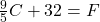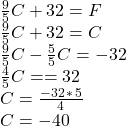## Two thermometers are calibrated, one in degrees Celsius and the other in degrees Fahrenheit. At what temperature are their readings nu

Question

Two thermometers are calibrated, one in degrees Celsius and the other in degrees Fahrenheit.
At what temperature are their readings numerically the same?

in progress 0
2 months 2021-07-23T13:59:45+00:00 1 Answers 5 views 0

The temperature is 233.15 K

Explanation:

Recall the formula to convert degree Celsius (C) into Fahrenheit (F):So if we want the value of degree C to be the same as the value of the degree F, we want the following: C = F

which replacing F with C on the right hand side of the equation above, allows us to solve for C:This means that -40°C = -40°F

And this temperature in Kelvin is:

-40°C + 273.15 = 233.15 K